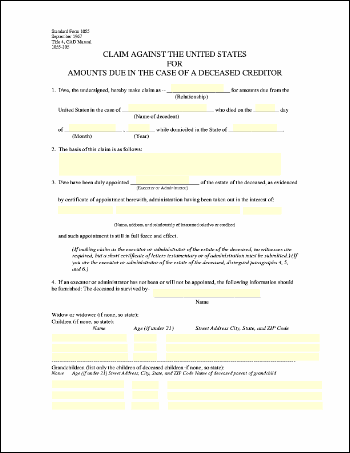eForm Stamp ExampleThis example shows how to stamp eForm fields into a document so that the values are indelibly marked.Src

 First, we create an ABCpdf Doc object and read in our template form. ```Set theDoc = Server.CreateObject("ABCpdf11.Doc") theDoc.Read "c:\mypdfs\form.pdf"```We set the values of selected fields, and then we stamp all field values into the document. ```theDoc.Form("Day") = "23" theDoc.Form("Month") = "February" theDoc.Form("Year") = "2005" theDoc.Form("State") = "Arizona" theDoc.Form.Stamp```Finally, we save. `theDoc.Save "c:\mypdfs\eformstamp.pdf"`Given the following document.form.pdf This is the kind of output you might expect.eformstamp.pdf